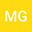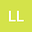A modification of a classical method to obtain Floquet exponents and solutions for linear periodic differential equations.
••Luis Pedro Lara
Instituto de Fisica Rosario
Author Profile## Abstract

We propose a modification of a method based on Fourier analysis to obtain the Floquet characteristic exponents for periodic homogeneous linear systems, which shows a high precision. This modification uses a variational principle to find the correct Floquet exponents among the solutions of an algebraic equation. Once we have these Floquet exponents, we determine explicit approximated solutions. We test our results on systems for which exact solutions are known to verify the accuracy of our method including one dimensional periodic potentials of interest in quantum physics. Using the equivalent linear system, we also study approximate solutions for homogeneous linear equations with periodic coefficients.
22 Sep 2022Submitted to Mathematical Methods in the Applied Sciences
23 Sep 2022Submission Checks Completed
23 Sep 2022Assigned to Editor
30 Sep 2022Reviewer(s) Assigned
24 Nov 2022Review(s) Completed, Editorial Evaluation Pending
25 Nov 2022Editorial Decision: Revise Major